$$\newcommand{\vecs}{\overset { \rightharpoonup} {\mathbf{#1}} }$$ $$\newcommand{\vecd}{\overset{-\!-\!\rightharpoonup}{\vphantom{a}\smash {#1}}}$$$$\newcommand{\id}{\mathrm{id}}$$ $$\newcommand{\Span}{\mathrm{span}}$$ $$\newcommand{\kernel}{\mathrm{null}\,}$$ $$\newcommand{\range}{\mathrm{range}\,}$$ $$\newcommand{\RealPart}{\mathrm{Re}}$$ $$\newcommand{\ImaginaryPart}{\mathrm{Im}}$$ $$\newcommand{\Argument}{\mathrm{Arg}}$$ $$\newcommand{\norm}{\| #1 \|}$$ $$\newcommand{\inner}{\langle #1, #2 \rangle}$$ $$\newcommand{\Span}{\mathrm{span}}$$ $$\newcommand{\id}{\mathrm{id}}$$ $$\newcommand{\Span}{\mathrm{span}}$$ $$\newcommand{\kernel}{\mathrm{null}\,}$$ $$\newcommand{\range}{\mathrm{range}\,}$$ $$\newcommand{\RealPart}{\mathrm{Re}}$$ $$\newcommand{\ImaginaryPart}{\mathrm{Im}}$$ $$\newcommand{\Argument}{\mathrm{Arg}}$$ $$\newcommand{\norm}{\| #1 \|}$$ $$\newcommand{\inner}{\langle #1, #2 \rangle}$$ $$\newcommand{\Span}{\mathrm{span}}$$$$\newcommand{\AA}{\unicode[.8,0]{x212B}}$$

## Parabolas

We will now study the graphs of quadratic equations in two variables with general form

$$y = ax^2 + bx + c, a \not= 0$$. $$a, b, c$$ are real numbers.

Parabola

All such graphs have a similar shape. The graph of a quadratic equation of this type Parabola is called a parabola and it will assume one of the following shapes.Vertex

The high point or low point of a parabola is called the vertex of the parabola.

## Constructing Graphs of Parabolas

We will construct the graph of a parabola by choosing several x-values, computing to find the corresponding y-values, plotting these ordered pairs, then drawing a smooth curve through them.

## Sample Set A

##### Example $$\PageIndex{1}$$

Graph $$y = x^2$$. Construct a table to exhibit several ordered pairs.

 $$x$$ $$y=x^2$$ 0 0 1 1 2 4 3 9 −1 1 −2 4 −3 9This is the most basic parabola. Although other parabolas may be wider, narrower, moved up or down, moved to the left or right, or inverted, they will all have this same basic shape. We will need to plot as many ordered pairs as necessary to ensure this basic shape.

##### Example $$\PageIndex{2}$$

Graph $$y = x^2 - 2$$. Construct a table of ordered pairs.

 $$x$$ $$y=x^2 -2$$ 0 −2 1 −1 2 2 3 7 −1 −1 −2 2 −3 7Notice that the graph of $$y = x^2 - 2$$ is precisely the graph of $$y = x^2$$ but translated 2 units down. Compare the equations of $$y = x^2$$ and $$y = x^2 - 2$$. Do you see what causes the 2 unit downward translation?

## Practice Set A

##### Practice Problem $$\PageIndex{1}$$

Use the idea suggested in Sample Set A to sketch (quickly and perhaps not perfectly accurately) the graphs of

$$y = x^2 + 1$$ and $$y = x^2 - 3$$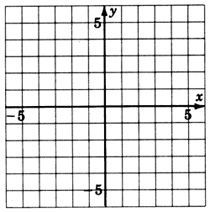## Sample Set B

##### Example $$\PageIndex{1}$$

Graph $$y = (x + 2)^2$$.

Do we expect the graph to be similar to the graph of $$y = x^2$$? Make a table of ordered pairs.

 $$x$$ $$y$$ 0 4 1 9 −1 1 −2 0 −3 1 −4 4Notice that the graph of $$y = (x + 2)^2$$ is precisely the graph of $$y = x^2$$ but translated 2 units to the left. The $$+2$$ inside the parentheses moves $$y = x^2$$ two units to the left. A negative value inside the parentheses makes a move to the right.

## Practice Set B

##### Practice Problem $$\PageIndex{1}$$

Use the idea suggested in Sample Set B to sketch the graphs of

$$y = (x-3)^2$$ and $$y = (x + 1)^2$$##### Practice Problem $$\PageIndex{3}$$

Graph $$y = (x-2)^2 + 1$$## Exercises

For the following problems, graph the quadratic equations.

##### Exercise $$\PageIndex{1}$$

$$y = x^2$$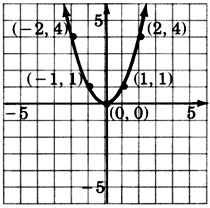##### Exercise $$\PageIndex{2}$$

$$y = -x^2$$##### Exercise $$\PageIndex{3}$$

$$y = (x-1)^2$$##### Exercise $$\PageIndex{4}$$

$$y = (x-2)^2$$##### Exercise $$\PageIndex{5}$$

$$y = (x + 3)^2$$##### Exercise $$\PageIndex{6}$$

$$y = (x + 3)^2$$##### Exercise $$\PageIndex{7}$$

$$y = (x + 1)^2$$##### Exercise $$\PageIndex{8}$$

$$y = x^2 - 3$$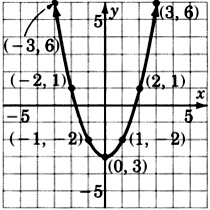##### Exercise $$\PageIndex{9}$$

$$y = x^2 - 1$$##### Exercise $$\PageIndex{10}$$

$$y = x^2 + 2$$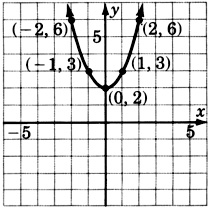##### Exercise $$\PageIndex{11}$$

$$y = x^2 + \dfrac{1}{2}$$##### Exercise $$\PageIndex{12}$$

$$y = x^2 - \dfrac{1}{2}$$##### Exercise $$\PageIndex{13}$$

$$y = -x^2 + 1$$ (compare with problem 2)##### Exercise $$\PageIndex{14}$$

$$y = -x^2 - 1$$ (compare with problem 1)##### Exercise $$\PageIndex{15}$$

$$y = (x-1)^2 - 1$$##### Exercise $$\PageIndex{16}$$

$$y = (x + 3)^2 + 2$$##### Exercise $$\PageIndex{17}$$

$$y = -(x + 1)^2$$##### Exercise $$\PageIndex{18}$$

$$y = -(x + 3)^2$$##### Exercise $$\PageIndex{19}$$

$$y = 2x^2$$##### Exercise $$\PageIndex{20}$$

$$y = 3x^2$$##### Exercise $$\PageIndex{21}$$

$$y = \dfrac{1}{2}x^2$$For the following problems, try to guess the quadratic equation that corresponds to the given graph.

##### Exercise $$\PageIndex{22}$$##### Exercise $$\PageIndex{23}$$$$y = (x-3)^2$$

##### Exercise $$\PageIndex{24}$$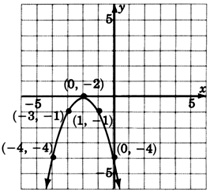##### Exercise $$\PageIndex{25}$$$$y = -(x + 3)^2 + 2$$

## Exercises For Review

##### Exercise $$\PageIndex{26}$$

Simplify and write $$(x^{-4}y^5)^{-3}(x^{-6}y^4)^2$$ so that only positive exponents appear.

##### Exercise $$\PageIndex{27}$$

Factor $$y^2 - y - 42$$

$$(y+6)(y−7)$$

##### Exercise $$\PageIndex{28}$$

Find the sum: $$\dfrac{2}{a - 3} + \dfrac{3}{a + 3} + \dfrac{18}{a^2 - 9}$$

##### Exercise $$\PageIndex{29}$$

Simplify $$\dfrac{2}{4 + \sqrt{5}}$$

$$\dfrac{8 - 2\sqrt{5}}{11}$$
##### Exercise $$\PageIndex{30}$$
Four is added to an integer and that sum is doubled. When this result is multiplied by the original integer, the product is $$-6$$. Find the integer.